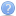column chart limit line
I've figured out a way of doing this but it's not nice:

I have 4 stacked ColumnSeries:
1 for the value.
1 for the gap between the value and the limit (with fill set to transparent).
1 for the limit (the value is always 1 to produce a line).
1 for the overflow

Then I just do a bit of logic like follows:

for(int i=0; i<data.Length; i++){
if(data[i].Value > data[i].Limit){
//set each column value accordingly
}else{
//set each column value accordingly
}
}
﻿